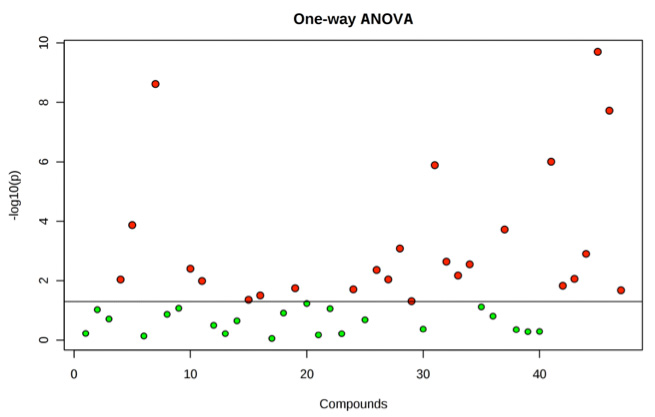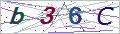Services

Online Inquiry

# ANOVA Analysis Service

Analysis of variance (ANOVA) is a collection of statistical models widely used to analyze the variation of the individual value from the mean value of the group, Such as "variation" among and between groups, was developed by Ronald Fisher. In the ANOVA method, the observed variance in a particular variable is partitioned into components attributable to different sources of variation. In its simplest form, ANOVA method provides a statistical test of whether or not the mean value of several groups are equal, and generalizes the t-test to compare more than two groups. ANOVAs are greatly useful for comparing three or more groups (or variables) for statistical significance. It is conceptually similar to multiple two-sample t-tests, but is more conservative that results in less type I error, and is therefore suited to a wide range of practical problems.

The normal-model based ANOVA approach assumes independence, normality and homogeneity of the variances of the residuals. However, randomization-based ANOVA analysis assumes only the homogeneity of the variances of the residuals and adopts the randomization procedure of the experiment. Both of the analyses request homoscedasticity, as an assumption for the normal-model analysis and as a result of randomization and additivity for the randomization-based analysis.ANOVA is applied in the analysis of comparative experiments. The statistical significance of the experiment depends on a ratio of two variances. This ratio is independent of quite a few possible alterations to the experimental observations: Adding or multiplying a constant to all observations does not change significance. So ANOVA statistical significance result is independent of scaling errors and constant bias as well as the units used in expressing observations.

The followings are three classified models used in the analysis of variance:

• Fixed-effects models
• Random-effects models
• Mixed-effects models

How to place an order:* For Research Use Only. Not for use in diagnostic procedures.
Our customer service representatives are available 24 hours a day, 7 days a week. Inquiry

Online Inquiry

Please submit a detailed description of your project. We will provide you with a customized project plan to meet your research requests. You can also send emails directly to for inquiries.

* Email
Phone
* Service & Products of Interest
Services Required and Project Description
* Verification Code